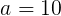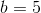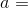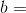# Computer Science : Variable Declarations

## Example Questions

### Example Question #1 : Variable Declarations

// ^ is Exclusive-Or (XOR) operator

x = x ^ y

y = x ^ y

x = x ^ y

What is the effect of the above snippet of code?

The values are switched.

None of the other answers is true.

The values are the same.

x is equal to y

The bits of x and y are negated.

The values are switched.

Explanation:

Consider two variables:(binary 1010) and(binary 0101). The exclusive or operator returns 1 in every bit that is different in the two numbers and returns 0 in every bit that is the same.

In order to switch the numbers, we must do this:^^^Continuing with the example,^would return 1111, because no bits are the same between the two numbers. Store this in the variable. Then 1111 ^ 0101 would return 1010. That result will be stored in. Finally, find the value of(1111) ^(1010) which is 0101, and store the result in variable. The values are now switched.

### Example Question #2 : Variable Declarations

Which of the following declares a String array of size i?  (Presume Java syntax.)

String[] s = new String[i];

None of the others

String s = new String[i];

String s[] = new String[i];

String[] s[i];

String[] s = new String[i];

Explanation:

For arrays, it is best to think about the array itself as being a type.  So, just as an int named x is declared:

int x;

And a String named s is declared:

String s;

You begin the declaration of a String array like this:

String[]

This is like saying, "What is coming next will be an array of String objects."

String[] s = new String[i];

This declares the new size as well, appropriately using the new operator.

### Example Question #3 : Variable Declarations

Which of the following code snippets declares an array of integers using an array literal?

int[] a = new int;

a = 42;

a = 26;

a = 134;

a = -13;

a = 45;

a = 234;

int[] a;

a = 42;

a = 26;

a = 134;

a = -13;

a = 45;

a = 234;

int[] a = {42,26,134,-13,45,234};

int a = {42,26,134,-13,45,234};

int[] a = {0:42,1:26,2:134,3:-13,4:45,5:234};

int[] a = {42,26,134,-13,45,234};

Explanation:

There are two things to pay attention to for this question.  First, you can make a simple array literal in Java by enclosing the list of array elements in a pair of curly braces.  Thus, the array literal

{42,26,134,-13,45,234}

is just fine!  However, you do not give the indices.  These are inferred.  (The first element is at 0, the second at 1, etc.)

Now, you just have to assign this to an array object.  Thus the following is wrong:

int a = {42,26,134,-13,45,234};

You need to have the [] to indicate that a is an array:

int[] a = {42,26,134,-13,45,234};

### Example Question #4 : Variable Declarations

What is the difference between double and int?

Int can only hold integer values, double can hold any number including decimals.

Int can hold all values whereas double can only store two-digit values.

There is no difference.

Int can hold real numbers and double can only hold imaginary numbers.

Int can hold positive numbers only and double can hold all numbers.

Int can only hold integer values, double can hold any number including decimals.

Explanation:

Int can only store integer values, whereas double can hold both integers and decimals, but at the same time uses more memory.

### Example Question #5 : Variable Declarations

int x=2;

double y=2.1;

float z=3.0;

int c=(x*y) + z;

What is the value of c

7.2

7

6.3

7.1

6

7

Explanation:

Remember that if a variable is declared as an integer, it can't have any decimals.

int c=(x*y) + z;

int c=(2*2.1)+3.0

From here, we do our math operations to solve, remembering to use the correct order of operations. Thus, start with the parentheses first then do the addition.

int c=4.2+3

int c= 4+3

int c=7

Any leftover fractions are cut off, so the answer is shortened to just 7.

### Example Question #6 : Variable Declarations

#include <iostream>

using namespace std;

int main()
{
string str("ComputerScience");
string mystr;
str = str + "SampleQuestionCS";
mystr = str.substr(7,8);

return 0;
}

What is the value of mystr after this code is run?

SampleQuestion

Science

rScience

ComputerS

QuestionCS

rScience

Explanation:

Two strings are created here: str and mystr.

In the 3rd line of main, str is concatenated with another string, making it even longer.

In the fourth line, the substr function is called. This function takes in 2 inputs, the starting index and the length of the string.

So, starting from index 7 of str, we get "r". Now, grabbing a total of 8 characters, including the "r", we get "rScience".

mystr="rScience".

### Example Question #7 : Variable Declarations

• Declare a string set to the the word cat.
• Declare a list of strings with the words hello, mama, and meow in it.
• Declare a hashmap of <string, string> <key, value> pairs with the word world having a value of earth.

String cat = "cat";

List<String> stringList = new ArrayList<String>();

stringList.add("hello");

stringList.add("mama");

stringList.add("meow");

HashMap<String, String> hashStrings = new HashMap<String, String>();

hashStrings.put("world", "earth");

List<String> stringList = new ArrayList<String>();

stringList.add("hello");

stringList.add("mama");

stringList.add("meow");

HashMap<String, String> hashStrings = new HashMap<String, String>();

hashStrings.put("world", "earth");

String cat = "cat";

List<String> stringList = new ArrayList<String>();

HashMap<String, String> hashStrings = new HashMap<String, String>();

hashStrings.put("world", "earth");

String cat;

List<String> stringList;

HashMap<String, String> hashStrings;

String cat = "cat";

List<String> stringList = new ArrayList<String>();

stringList.add("hello");

stringList.add("mama");

stringList.add("meow");

HashMap<String, String> hashStrings = new HashMap<String, String>();

hashStrings.put("world", "earth");

Explanation:

The correct answer defines all of the variables specified in the prompt. The answer with just the variable definitons is incorrect because it does not specify the declarations in the prompt. One the answers does not have the first variable defined so it is incorrect. And the final answer does not have the List variable declared, so it is also incorrect.

### Example Question #8 : Variable Declarations

What are the 3 different manners (in terms of accessibilitiy) in which you can declare a variable and what are the differences between the 3?

Public: accessible to everyone
Private: accessible to members of the class
Protected:accessible to classes in the same package and subclasses

Public: accessible to everyone
Private: accessible to only members of the method
Protected: accessible to subclass

Public: accessible to that sole class

Private: accessible to the method or instance in which it was called

Protected: acts as the default setting

Public: accessible to everyone
Private: accessible to members of the class
Protected:accessible to classes in the same package and subclasses

Explanation:

Public is accessible to everyone regardless if the two classes are in the same package or not.

Protected: Only allows access of those extended are packaging.

Private: Its meant to keep private in order for only members of that specific class to see it.

### Example Question #9 : Variable Declarations

Which of these produce a typecasting error?

1) float f = double d;

2) double d = int i;

3) int i = byte b;

4) long l = short s;

int i = byte b;

double d = int i;

None of the answers are wrong.

long l = short s;

float f = double d;